Скачать презентацию Introduction to Functional Programming and ML CS 331

d738519d9e77a9c72f8489c401450252.ppt

• Количество слайдов: 26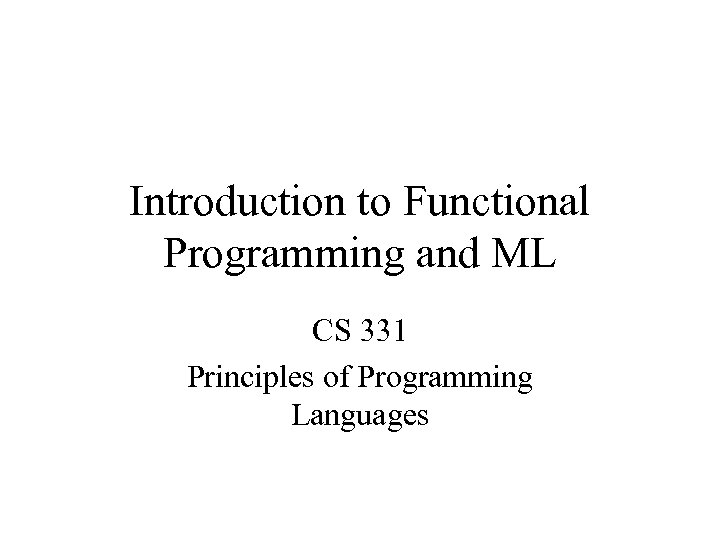Introduction to Functional Programming and ML CS 331 Principles of Programming LanguagesHow to do screen captures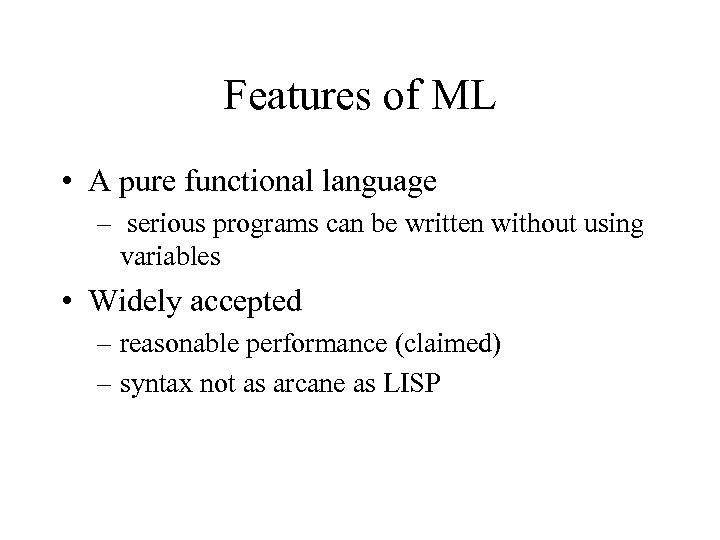Features of ML • A pure functional language – serious programs can be written without using variables • Widely accepted – reasonable performance (claimed) – syntax not as arcane as LISPIn these slides, • We use Standard ML of New Jersey • Runs on PCs, and lots of other platforms • See the ML API documentation at http: //cm. belllabs. com/cm/cs/what/smlnj/doc/basis/pages/sml-stdbasis. html • For information about ML on the web, see – http: //cm. bell-labs. com/cm/cs/what/smlnj/index. html – http: //foxnet. cs. cmu. edu/sml. html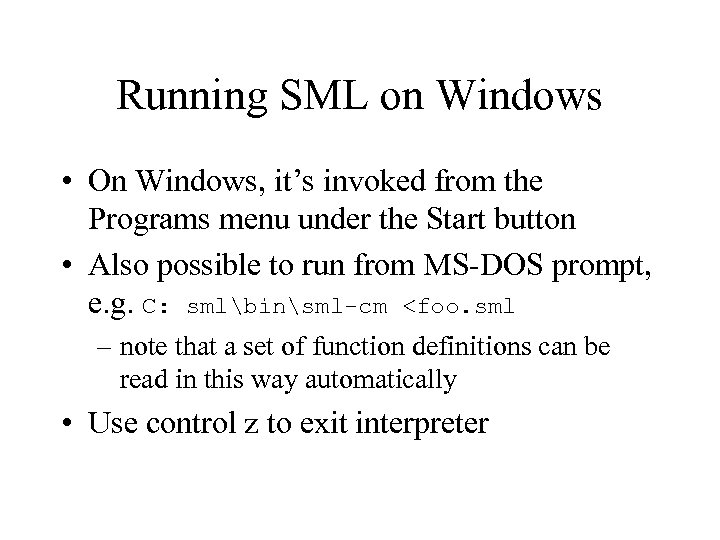Running SML on Windows • On Windows, it’s invoked from the Programs menu under the Start button • Also possible to run from MS-DOS prompt, e. g. C: smlbinsml-cm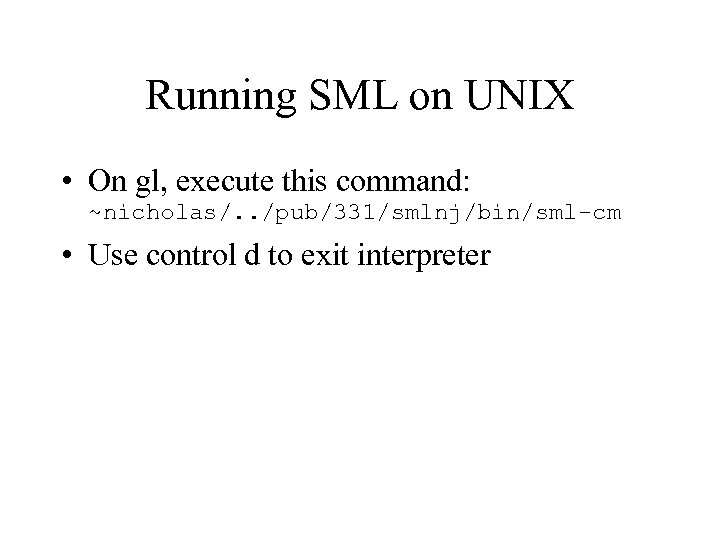Running SML on UNIX • On gl, execute this command: ~nicholas/. . /pub/331/smlnj/bin/sml-cm • Use control d to exit interpreter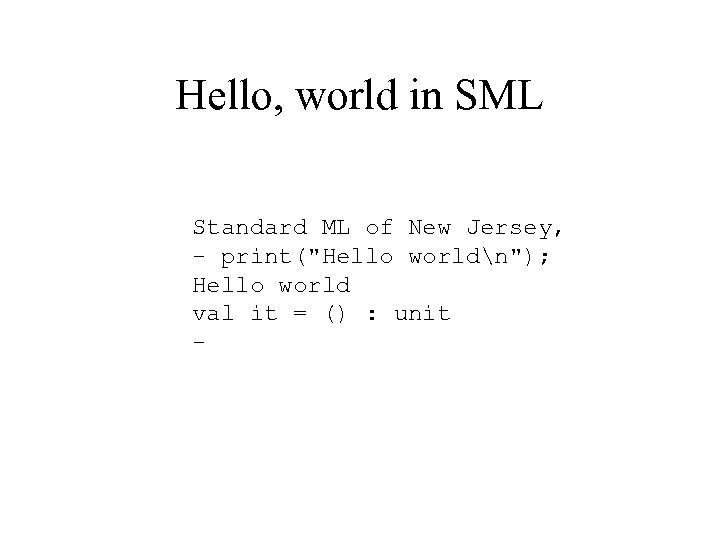Hello, world in SML Standard ML of New Jersey, - print("Hello worldn"); Hello world val it = () : unit -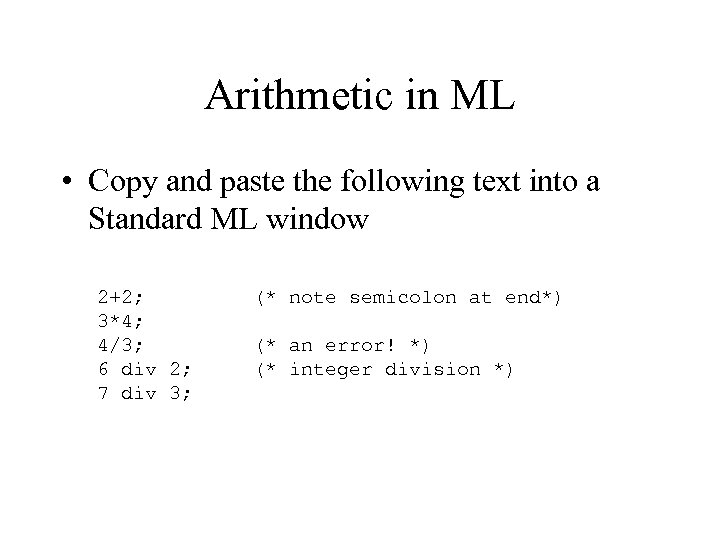Arithmetic in ML • Copy and paste the following text into a Standard ML window 2+2; 3*4; 4/3; 6 div 2; 7 div 3; (* note semicolon at end*) (* an error! *) (* integer division *)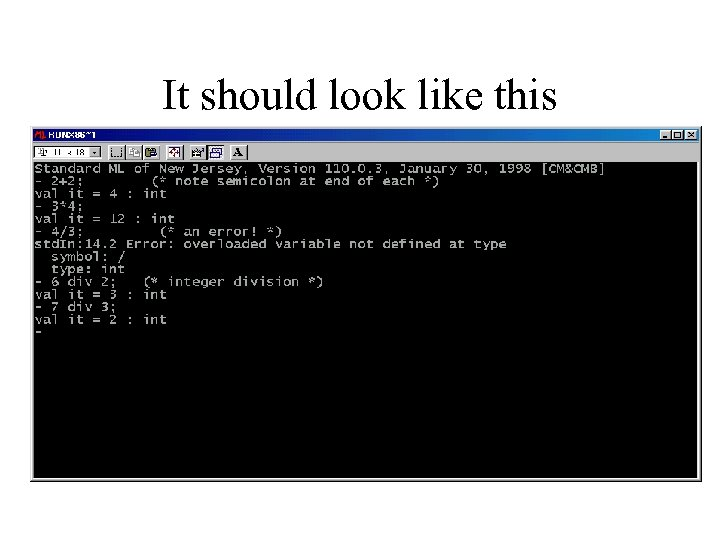It should look like this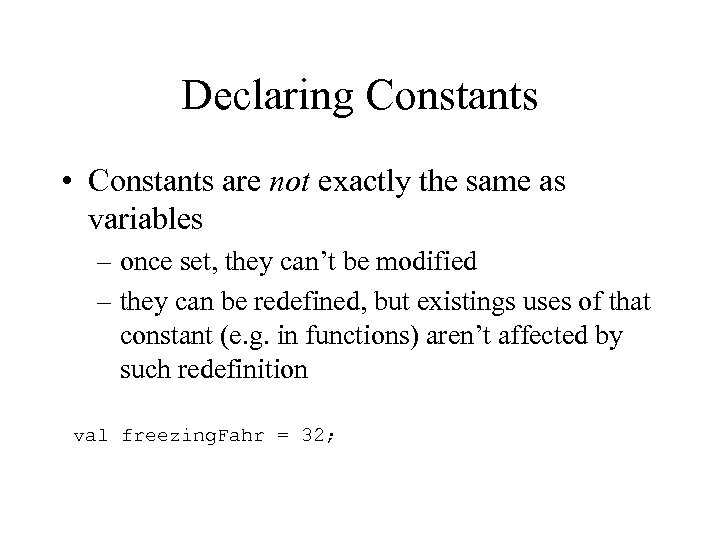Declaring Constants • Constants are not exactly the same as variables – once set, they can’t be modified – they can be redefined, but existings uses of that constant (e. g. in functions) aren’t affected by such redefinition val freezing. Fahr = 32;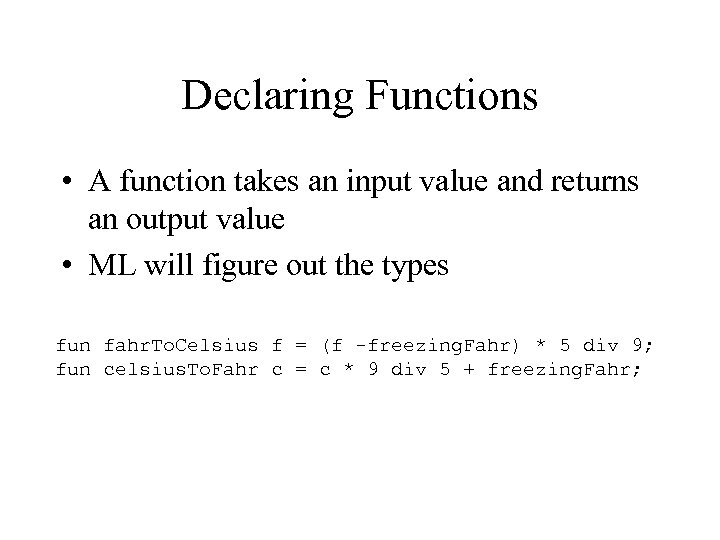Declaring Functions • A function takes an input value and returns an output value • ML will figure out the types fun fahr. To. Celsius f = (f -freezing. Fahr) * 5 div 9; fun celsius. To. Fahr c = c * 9 div 5 + freezing. Fahr;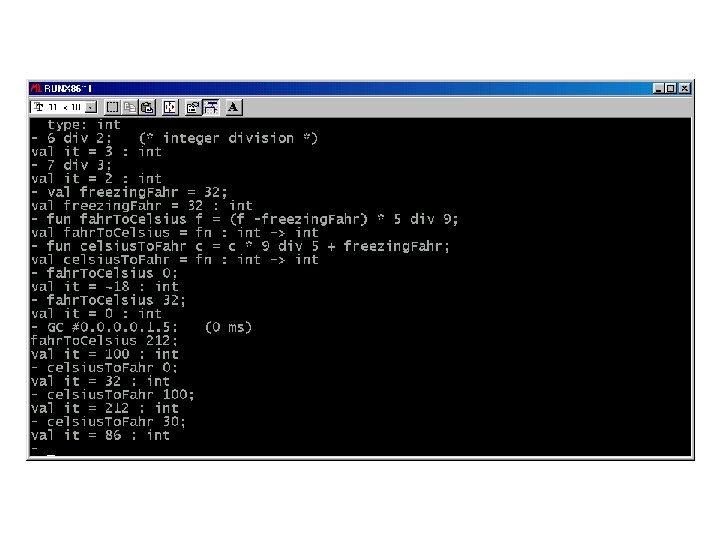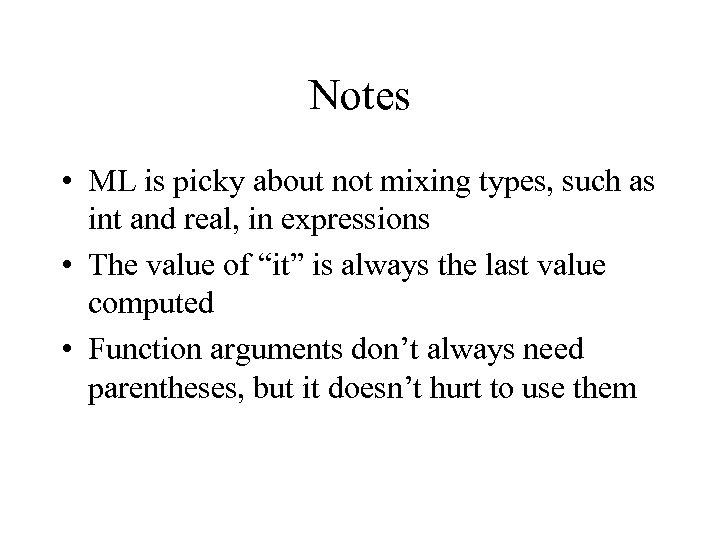Notes • ML is picky about not mixing types, such as int and real, in expressions • The value of “it” is always the last value computed • Function arguments don’t always need parentheses, but it doesn’t hurt to use them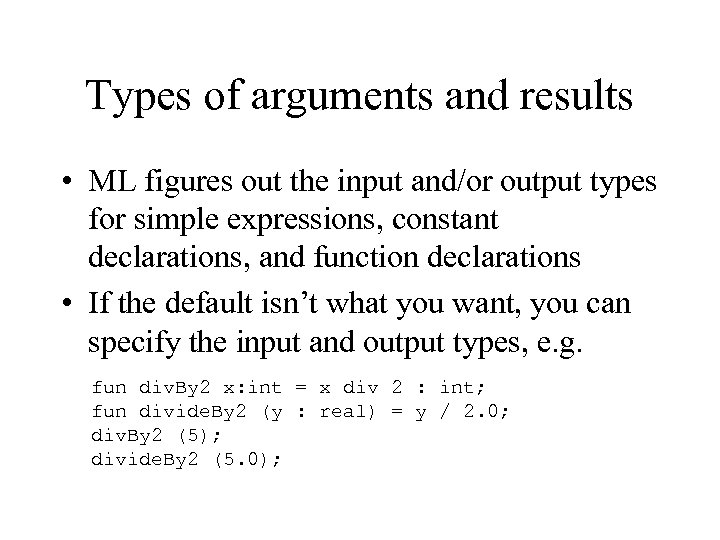Types of arguments and results • ML figures out the input and/or output types for simple expressions, constant declarations, and function declarations • If the default isn’t what you want, you can specify the input and output types, e. g. fun div. By 2 x: int = x div 2 : int; fun divide. By 2 (y : real) = y / 2. 0; div. By 2 (5); divide. By 2 (5. 0);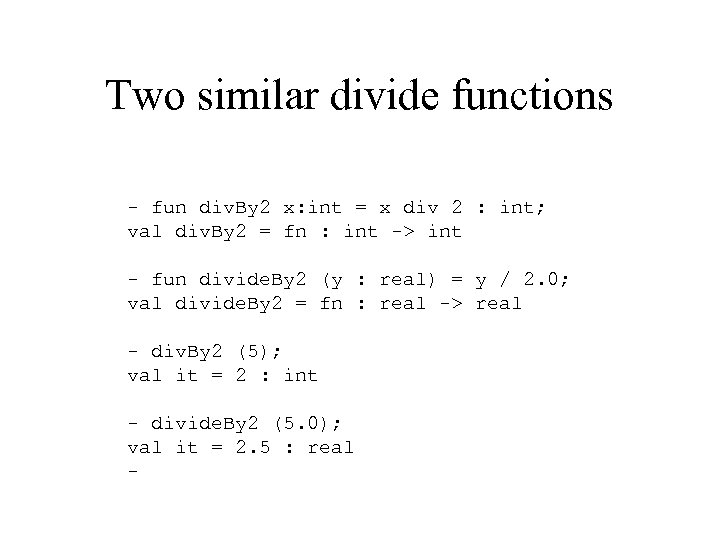Two similar divide functions - fun div. By 2 x: int = x div 2 : int; val div. By 2 = fn : int -> int - fun divide. By 2 (y : real) = y / 2. 0; val divide. By 2 = fn : real -> real - div. By 2 (5); val it = 2 : int - divide. By 2 (5. 0); val it = 2. 5 : real -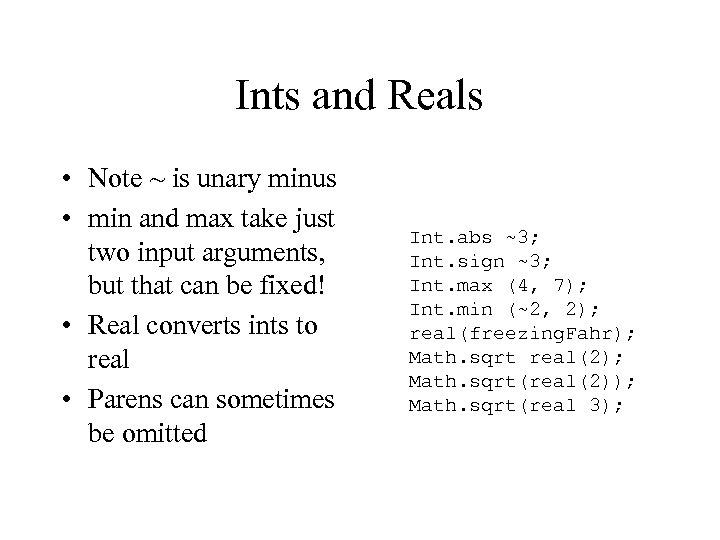Ints and Reals • Note ~ is unary minus • min and max take just two input arguments, but that can be fixed! • Real converts ints to real • Parens can sometimes be omitted Int. abs ~3; Int. sign ~3; Int. max (4, 7); Int. min (~2, 2); real(freezing. Fahr); Math. sqrt real(2); Math. sqrt(real(2)); Math. sqrt(real 3);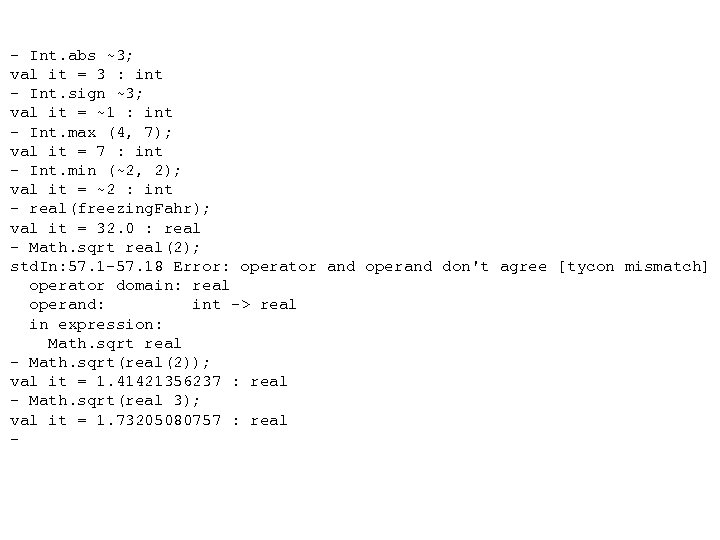- Int. abs ~3; val it = 3 : int - Int. sign ~3; val it = ~1 : int - Int. max (4, 7); val it = 7 : int - Int. min (~2, 2); val it = ~2 : int - real(freezing. Fahr); val it = 32. 0 : real - Math. sqrt real(2); std. In: 57. 1 -57. 18 Error: operator and operand don't agree [tycon mismatch] operator domain: real operand: int -> real in expression: Math. sqrt real - Math. sqrt(real(2)); val it = 1. 41421356237 : real - Math. sqrt(real 3); val it = 1. 73205080757 : real -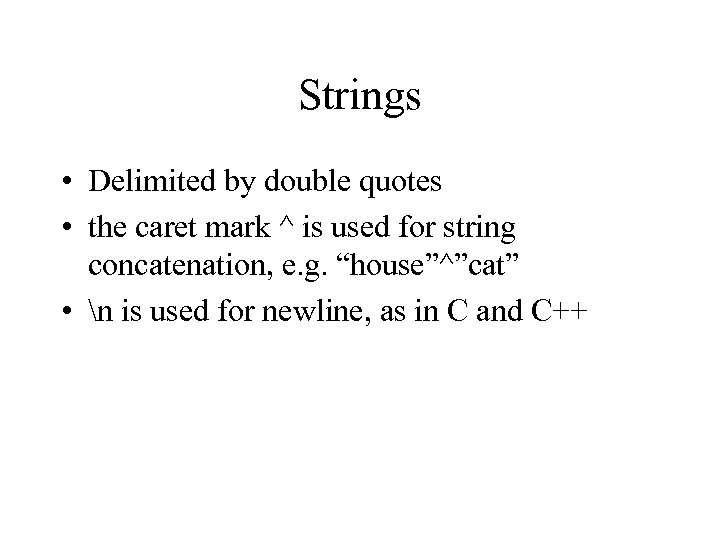Strings • Delimited by double quotes • the caret mark ^ is used for string concatenation, e. g. “house”^”cat” • n is used for newline, as in C and C++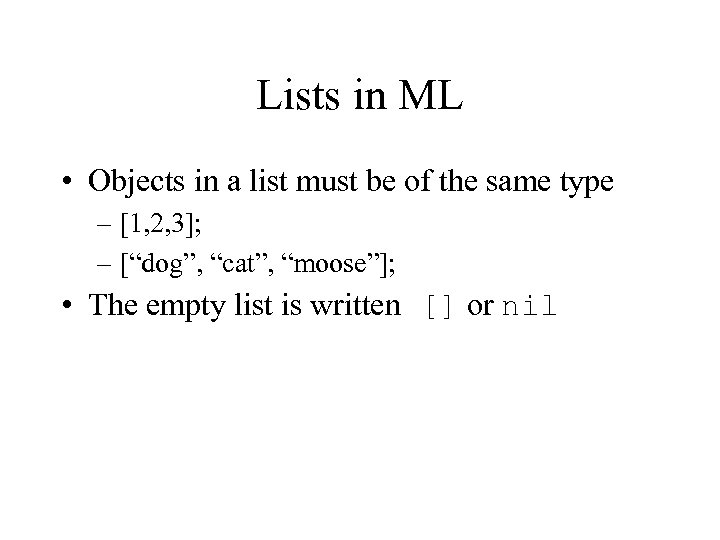Lists in ML • Objects in a list must be of the same type – [1, 2, 3]; – [“dog”, “cat”, “moose”]; • The empty list is written [] or nil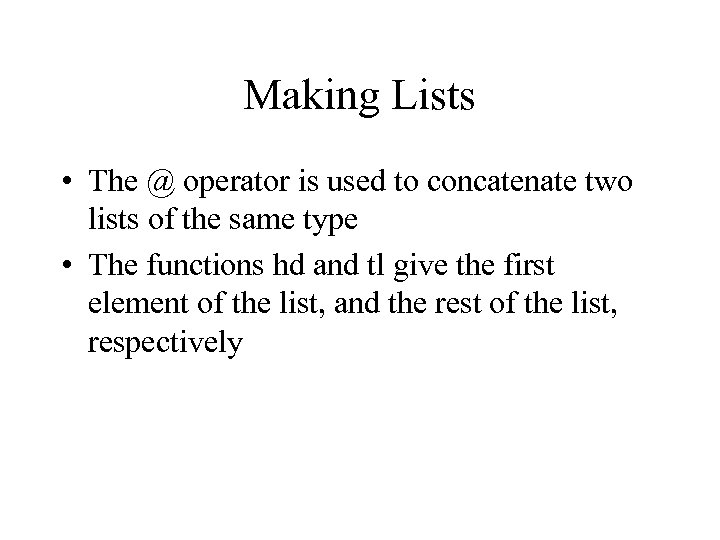Making Lists • The @ operator is used to concatenate two lists of the same type • The functions hd and tl give the first element of the list, and the rest of the list, respectively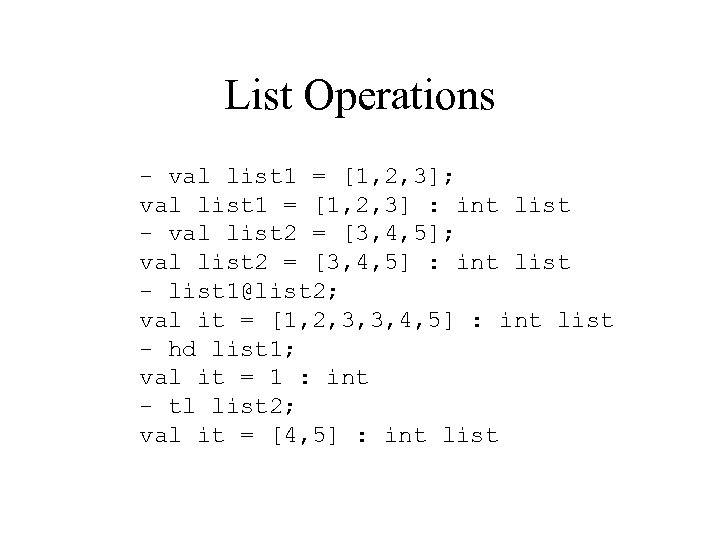List Operations - val list 1 = [1, 2, 3]; val list 1 = [1, 2, 3] : int list - val list 2 = [3, 4, 5]; val list 2 = [3, 4, 5] : int list - list [email protected] 2; val it = [1, 2, 3, 3, 4, 5] : int list - hd list 1; val it = 1 : int - tl list 2; val it = [4, 5] : int list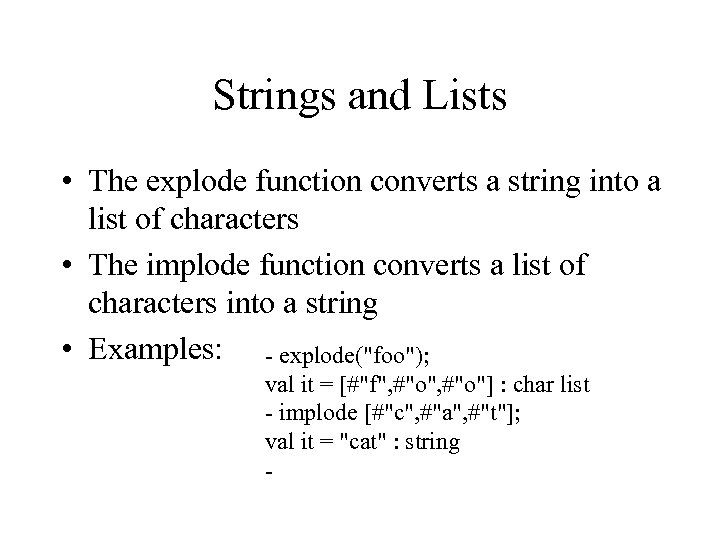Strings and Lists • The explode function converts a string into a list of characters • The implode function converts a list of characters into a string • Examples: - explode("foo"); val it = [#"f", #"o"] : char list - implode [#"c", #"a", #"t"]; val it = "cat" : string -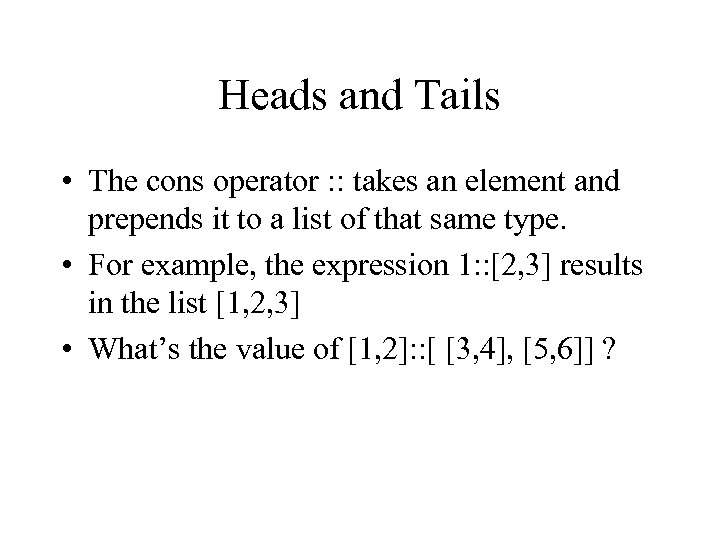Heads and Tails • The cons operator : : takes an element and prepends it to a list of that same type. • For example, the expression 1: : [2, 3] results in the list [1, 2, 3] • What’s the value of [1, 2]: : [ [3, 4], [5, 6]] ?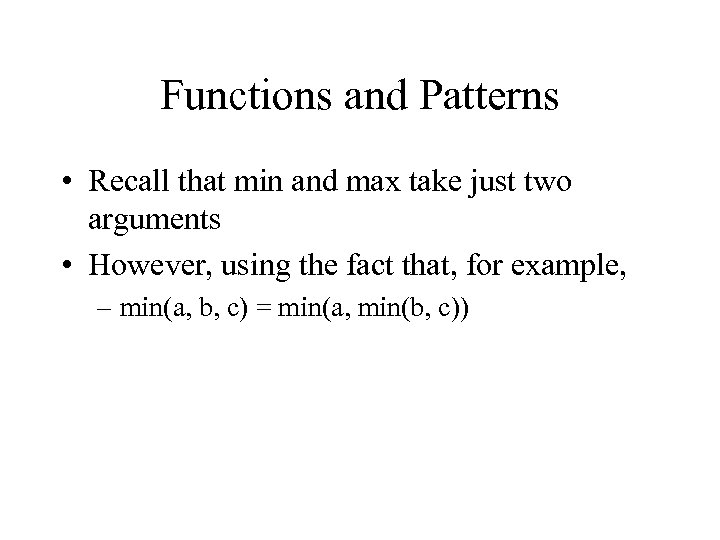Functions and Patterns • Recall that min and max take just two arguments • However, using the fact that, for example, – min(a, b, c) = min(a, min(b, c))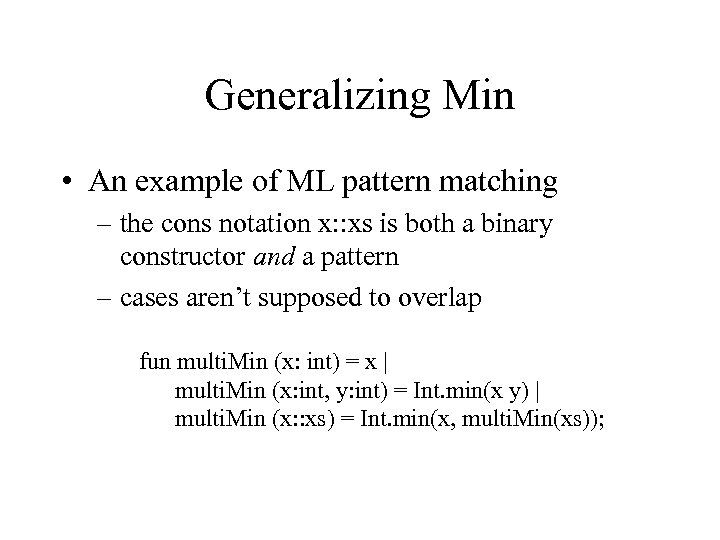Generalizing Min • An example of ML pattern matching – the cons notation x: : xs is both a binary constructor and a pattern – cases aren’t supposed to overlap fun multi. Min (x: int) = x | multi. Min (x: int, y: int) = Int. min(x y) | multi. Min (x: : xs) = Int. min(x, multi. Min(xs));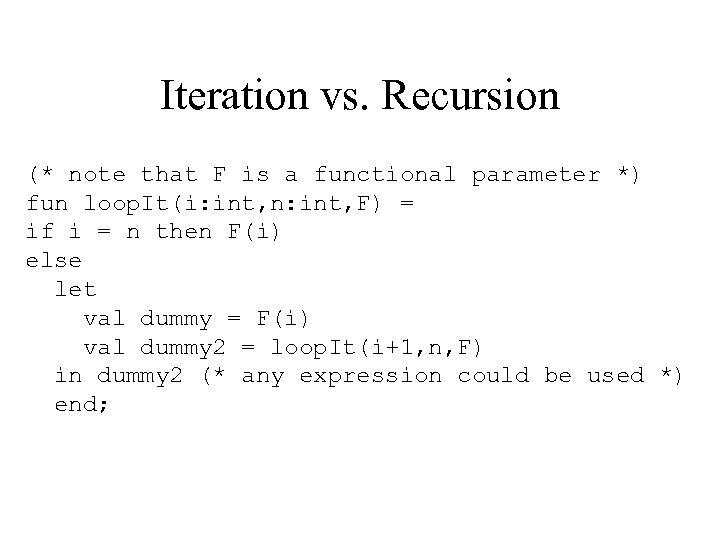Iteration vs. Recursion (* note that F is a functional parameter *) fun loop. It(i: int, n: int, F) = if i = n then F(i) else let val dummy = F(i) val dummy 2 = loop. It(i+1, n, F) in dummy 2 (* any expression could be used *) end;